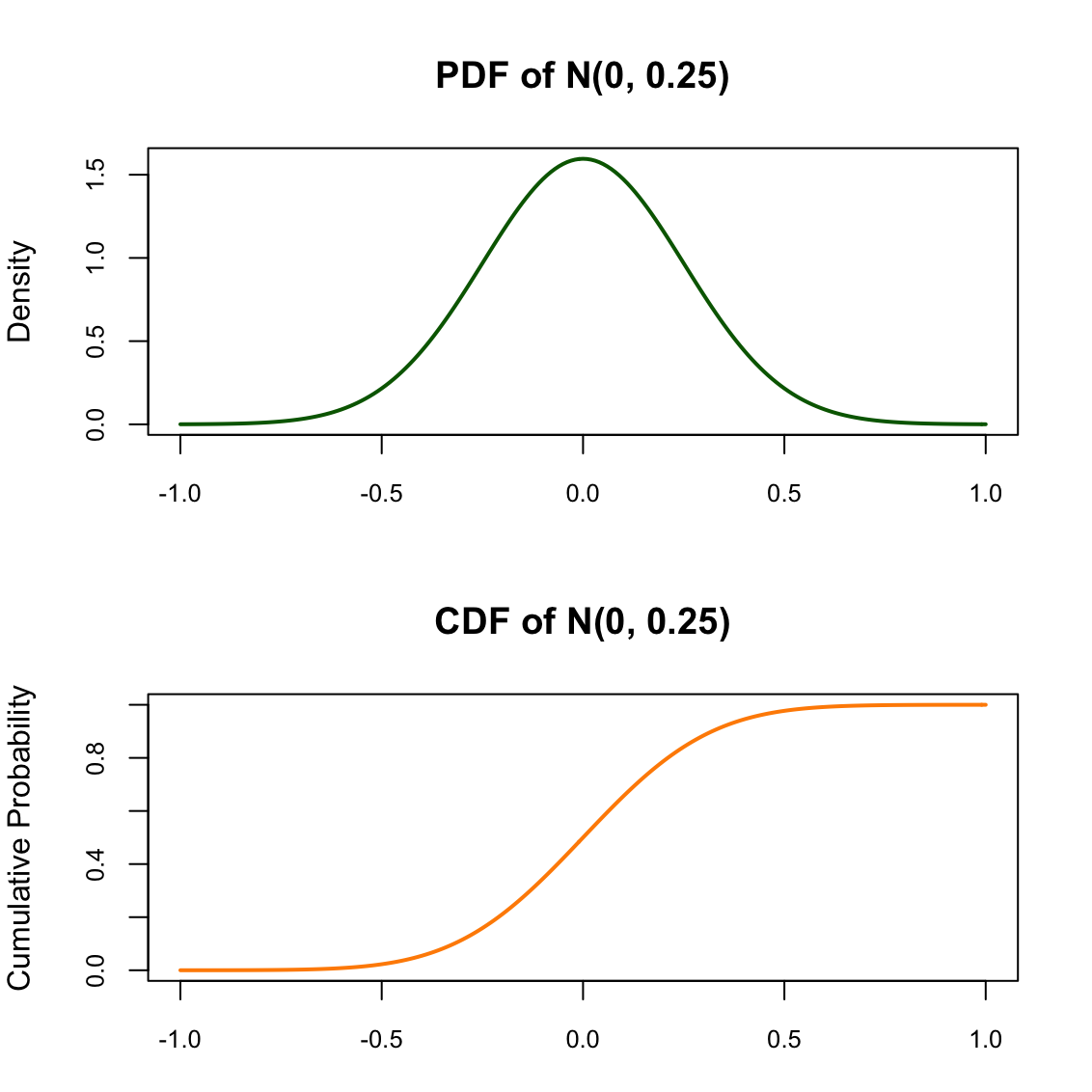# Honey, my pdf is greater than 1!

This is a confusing concept because of the density function for discrete distributions. These have a probability mass function, not a probability density function, and the interpretation is what we’re accustomed to. If we evalute the PMF at any point, $X = x$, that value corresponds to the probability of us observing $X = x$. So if we toss a coin, then $p(X = \text{Head})$ is the probability of observing a head.

When we try to extend this logic to probability density functions, weird things happen. Because PDF’s describe continuous distributions, the probability that we observe the exact value $X = x$ is 0 (as you have probably heard 100 times). So evaluating the PDF at a particular point doesn’t correspond to a probability. Then what does it correspond to? And how do we interpret it?

It helps to take a step back. The PDF, $f(x)$, is the derivative of the cumulative distribution function, $F(x)$. So at any given point, $X = x$ in the support, in order to get the value of the PDF, we can differentate the CDF and evaluate it at $X = x$. If you let this marinate for a bit, things should start to clear up and it becomes less natural to interpret the value as a probability.So it’s not a probability, what is it? Well, we could interpret it similar to how we interpret gradients. A specific point in a function doesn’t have a gradient. However, we can take a really small slice of the function and find the gradient there, then make claims about the gradient in that region. So, now we know that higher values of this derivative correspond to regions that are more likely, and vice versa for smaller values of the derviative.